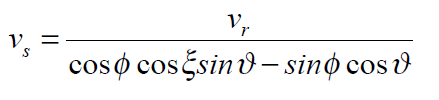# LOS to horizontal displacement

Hi all,

I want to know how can I convert the LOS displacement to horizontal displacement in glacier. I know the tool is not available yet in SNAP. But if any one has done this by using the LOS displacement map generated from SNAP please share on how is it possible?

2 Likes

to my understanding, this depends on the movement direction of the glacier and the pass (ascending or descending) of the image.
I don’t think it is possible in SNAP without generating whole DEMs of the glacier at two times (which isn’t less challenging).

1 Like

Often the assumption is surface-parallel flow so a DEM is needed to derive that as well.

thank you… yes, i think using an external DEM and assuming surface parallel flow, horizontal displacement can be calculated… i just know the theory… if someone who has done practically would be of help…

So for external DEM, if i use SRTM DEM (even though precise DEM would be aspired) do you know how I can derive the horizontal displacement?

The formula to be used is:where ξ is the angle of the flow direction, φ of the surface slope and ϑ is the local incidence angle respect the range
direction.

I do not know how to derive the term ξ. Any help shall be appreciated.

1 Like

You need to determine the flow direction vector “by hand” by examining the glacier geometry and then assume either horizontal or surface-parallel flow.

thank you so much… so its going to be only in the line that I draw by hand… like the centerline of the glacier… than I should make the assumption and make the calculation??